APPENDIX A REVIEW OF LINEAR ALGEBRA APPENDIX B

• Slides: 32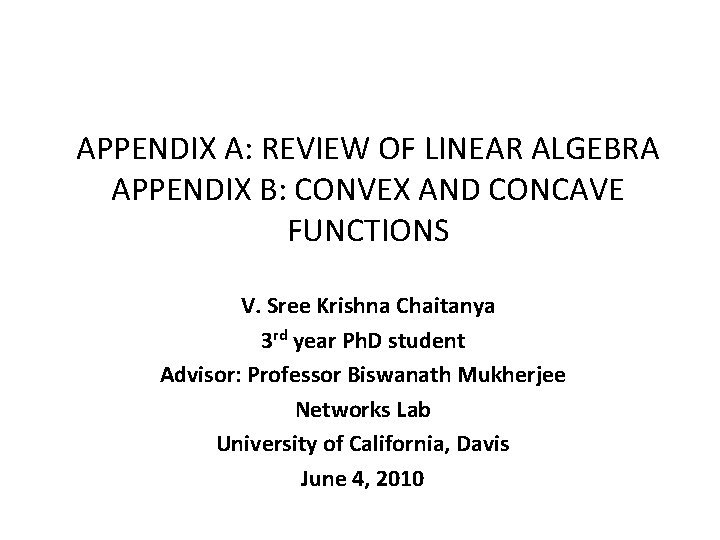APPENDIX A: REVIEW OF LINEAR ALGEBRA APPENDIX B: CONVEX AND CONCAVE FUNCTIONS V. Sree Krishna Chaitanya 3 rd year Ph. D student Advisor: Professor Biswanath Mukherjee Networks Lab University of California, Davis June 4, 2010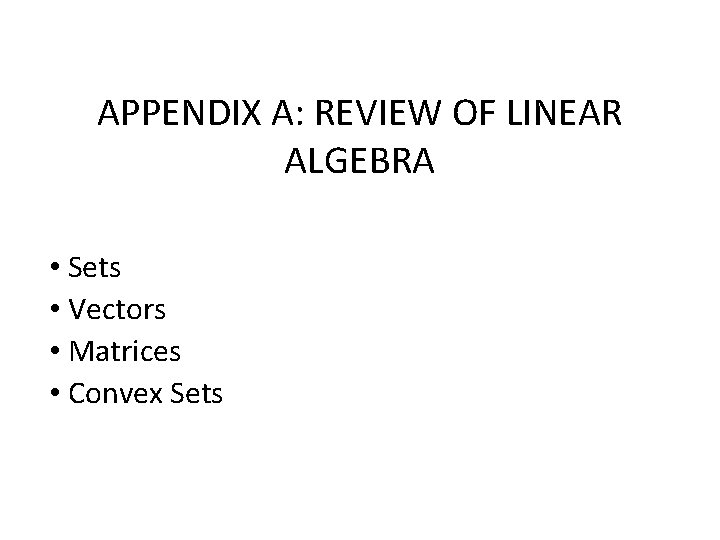APPENDIX A: REVIEW OF LINEAR ALGEBRA • Sets • Vectors • Matrices • Convex SetsSet Theory • Set is a well-defined collection of things. • Example: set S ={ x|x>= 0}, is a set of all non-negative numbers. • x = 2 is an element of S. Denoted as 2 Є S. • Union of two sets: is another Set. • R = {x|x Є P or x Є Q or both} • Intersection of two sets: is another set. • R = {x|x Є P and x Є Q} • Subset: Denoted as P Q, every element P is in Q. • Disjoint Sets: No elements in common. • Empty Set: Φ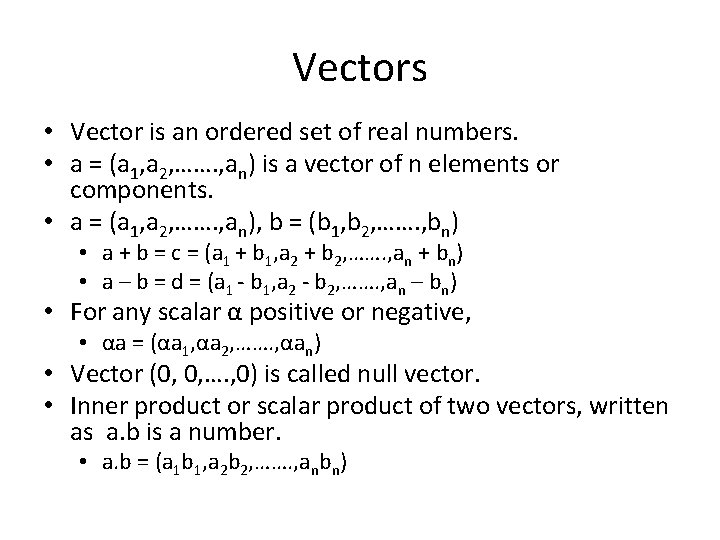Vectors • Vector is an ordered set of real numbers. • a = (a 1, a 2, ……. , an) is a vector of n elements or components. • a = (a 1, a 2, ……. , an), b = (b 1, b 2, ……. , bn) • a + b = c = (a 1 + b 1, a 2 + b 2, ……. , an + bn) • a – b = d = (a 1 - b 1, a 2 - b 2, ……. , an – bn) • For any scalar α positive or negative, • αa = (αa 1, αa 2, ……. , αan) • Vector (0, 0, …. , 0) is called null vector. • Inner product or scalar product of two vectors, written as a. b is a number. • a. b = (a 1 b 1, a 2 b 2, ……. , anbn)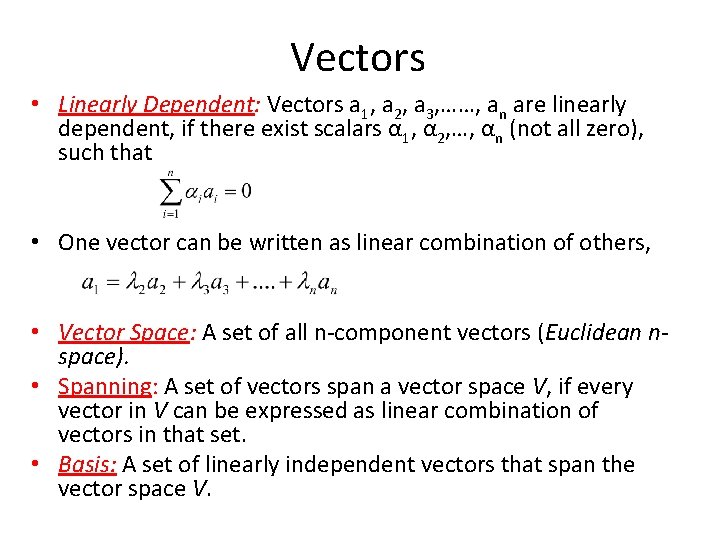Vectors • Linearly Dependent: Vectors a 1, a 2, a 3, ……, an are linearly dependent, if there exist scalars α 1, α 2, …, αn (not all zero), such that • One vector can be written as linear combination of others, • Vector Space: A set of all n-component vectors (Euclidean nspace). • Spanning: A set of vectors span a vector space V, if every vector in V can be expressed as linear combination of vectors in that set. • Basis: A set of linearly independent vectors that span the vector space V.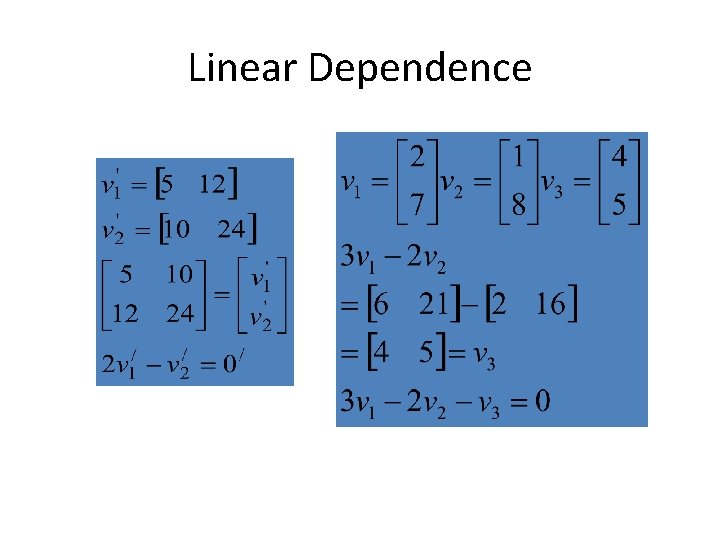Linear Dependence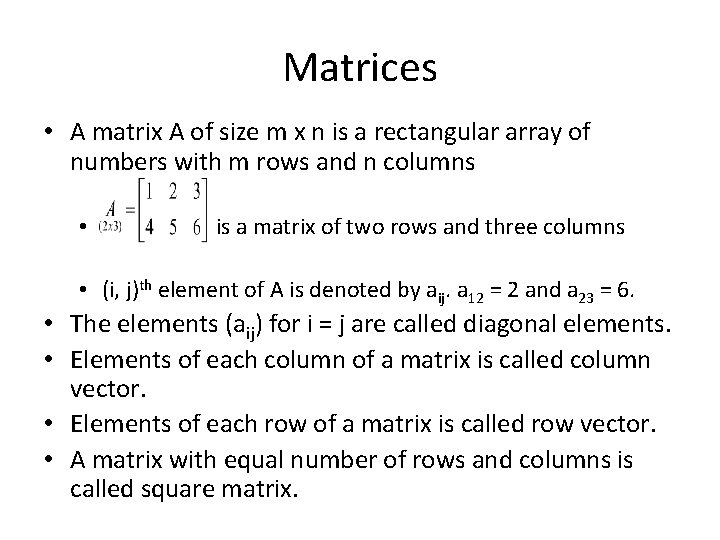Matrices • A matrix A of size m x n is a rectangular array of numbers with m rows and n columns • is a matrix of two rows and three columns • (i, j)th element of A is denoted by aij. a 12 = 2 and a 23 = 6. • The elements (aij) for i = j are called diagonal elements. • Elements of each column of a matrix is called column vector. • Elements of each row of a matrix is called row vector. • A matrix with equal number of rows and columns is called square matrix.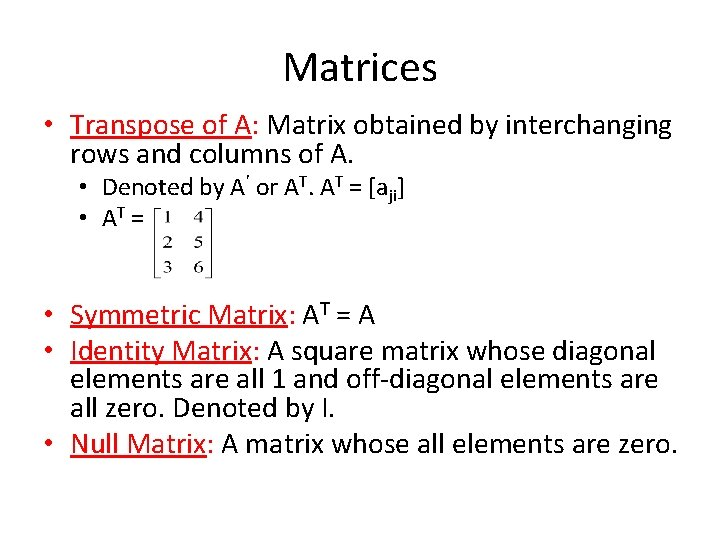Matrices • Transpose of A: Matrix obtained by interchanging rows and columns of A. • Denoted by A’ or AT. AT = [aji] • AT = • Symmetric Matrix: AT = A • Identity Matrix: A square matrix whose diagonal elements are all 1 and off-diagonal elements are all zero. Denoted by I. • Null Matrix: A matrix whose all elements are zero.Matrix Operations • Sum or difference of two matrices A and B is a matrix C. • C = A B, cij = aij bij • Product AB is defined if and only if, the no. of columns of A is equal to no. of rows of B • If A is an m x n matrix and B is an n x r matrix, then AB = C is a matrix of size m x r. • The (i, j)th element of C is given by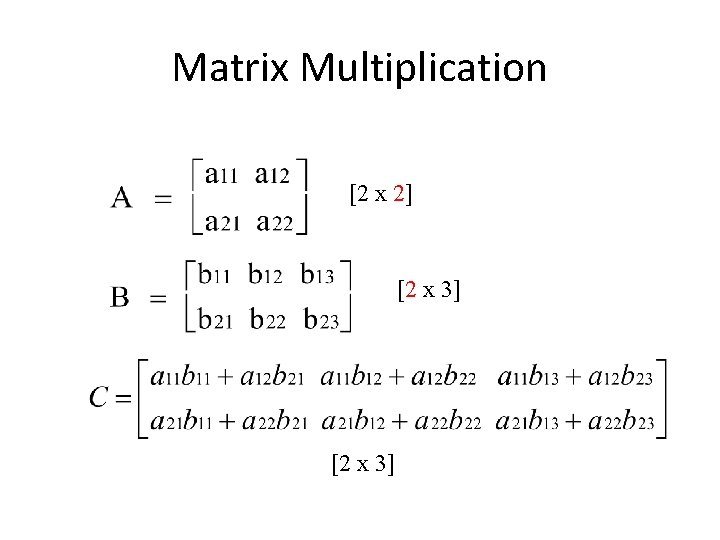Matrix Multiplication [2 x 2] [2 x 3]Matrix Operations • • • For any scalar α, αA = [αaij] (A + B) + C = A + (B + C) A+B=B+A (A+B)C = AC + BC AB ≠ BA (AB)C = A(BC) IA = AI = A (A + B)T = AT + BT (AB)T = BTAT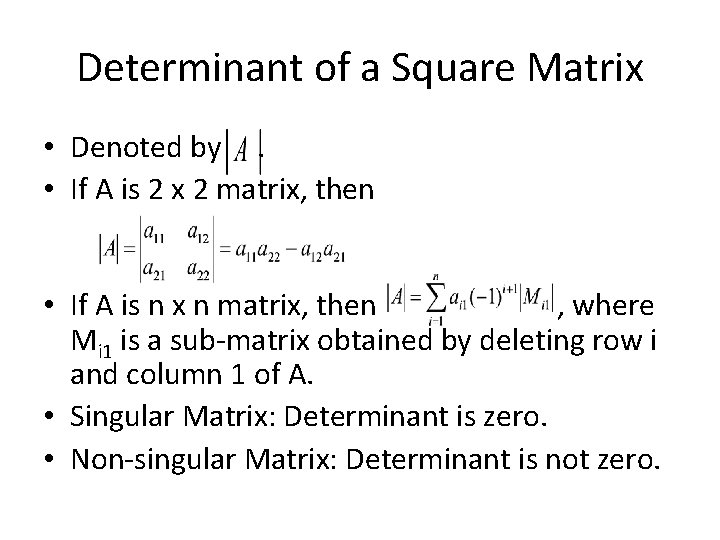Determinant of a Square Matrix • Denoted by. • If A is 2 x 2 matrix, then • If A is n x n matrix, then , where Mi 1 is a sub-matrix obtained by deleting row i and column 1 of A. • Singular Matrix: Determinant is zero. • Non-singular Matrix: Determinant is not zero.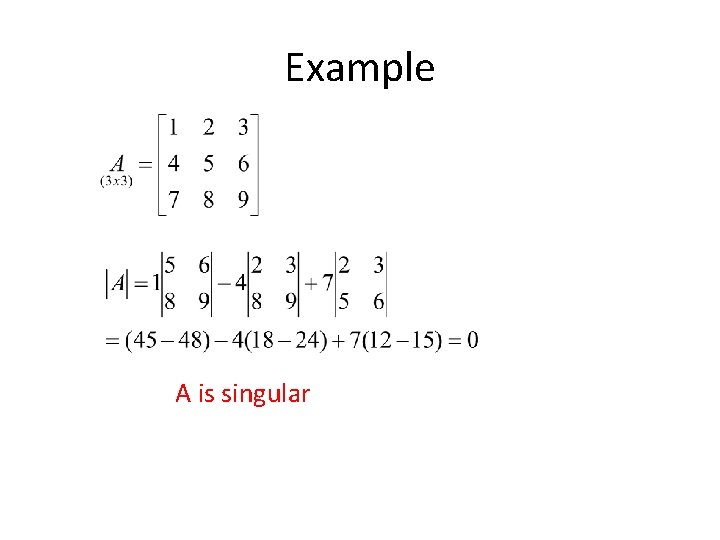Example A is singularInverse of a Matrix • The inverse of a matrix A is denoted by A-1. • It is defined only for non-singular square matrices. • AA-1 = I (identity matrix) • Unknown set of values x can be found by inverse of matrix AExample Inverse Matrix: In words: 2 x 2 • Take the original matrix. • Switch a and d. • Change the signs of b and c. • Multiply the new matrix by 1 over the determinant of the original matrix.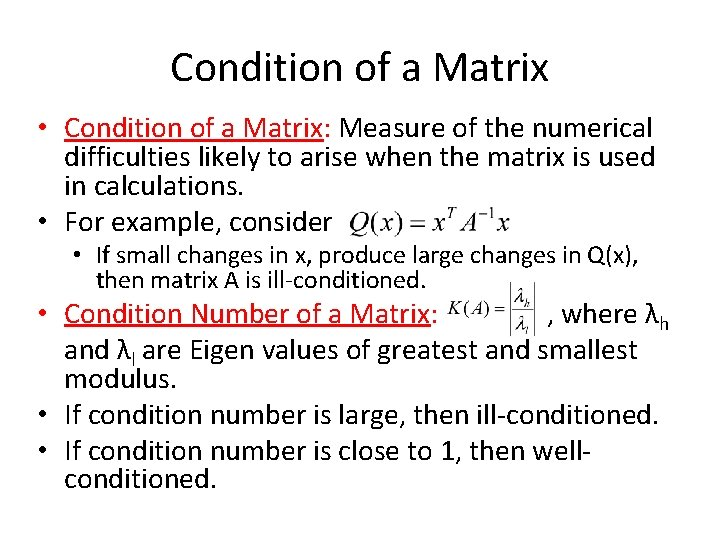Condition of a Matrix • Condition of a Matrix: Measure of the numerical difficulties likely to arise when the matrix is used in calculations. • For example, consider • If small changes in x, produce large changes in Q(x), then matrix A is ill-conditioned. • Condition Number of a Matrix: , where λh and λl are Eigen values of greatest and smallest modulus. • If condition number is large, then ill-conditioned. • If condition number is close to 1, then wellconditioned.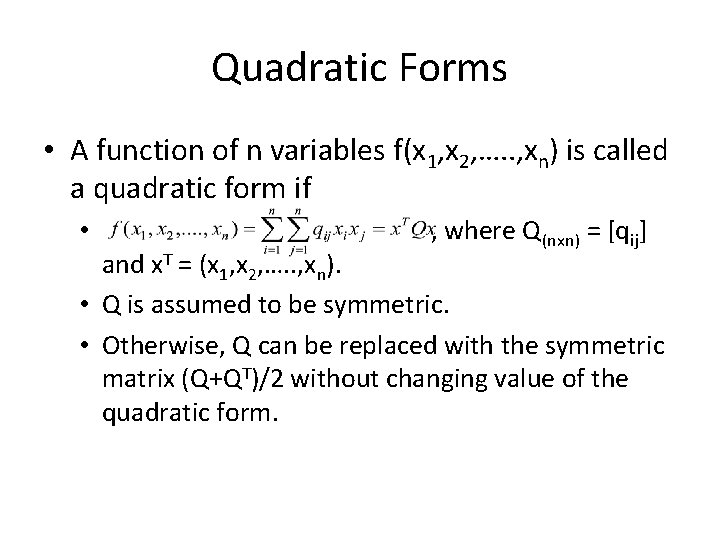Quadratic Forms • A function of n variables f(x 1, x 2, …. . , xn) is called a quadratic form if • , where Q(nxn) = [qij] and x. T = (x 1, x 2, …. . , xn). • Q is assumed to be symmetric. • Otherwise, Q can be replaced with the symmetric matrix (Q+QT)/2 without changing value of the quadratic form.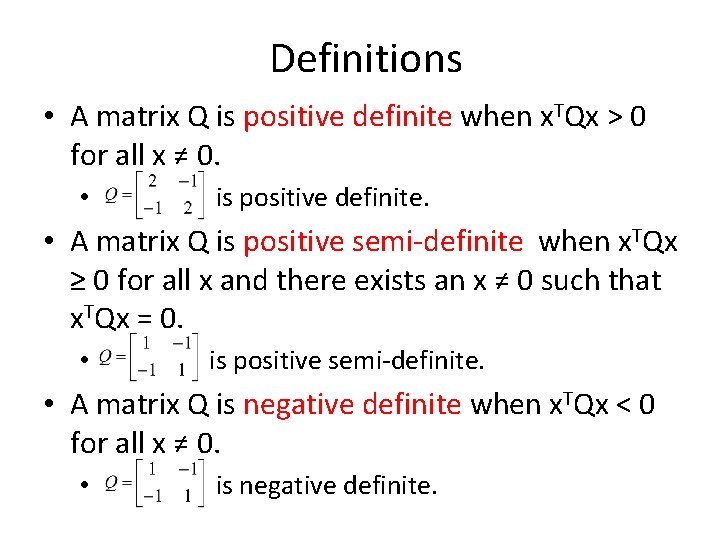Definitions • A matrix Q is positive definite when x. TQx > 0 for all x ≠ 0. • is positive definite. • A matrix Q is positive semi-definite when x. TQx ≥ 0 for all x and there exists an x ≠ 0 such that x. TQx = 0. • is positive semi-definite. • A matrix Q is negative definite when x. TQx < 0 for all x ≠ 0. • is negative definite.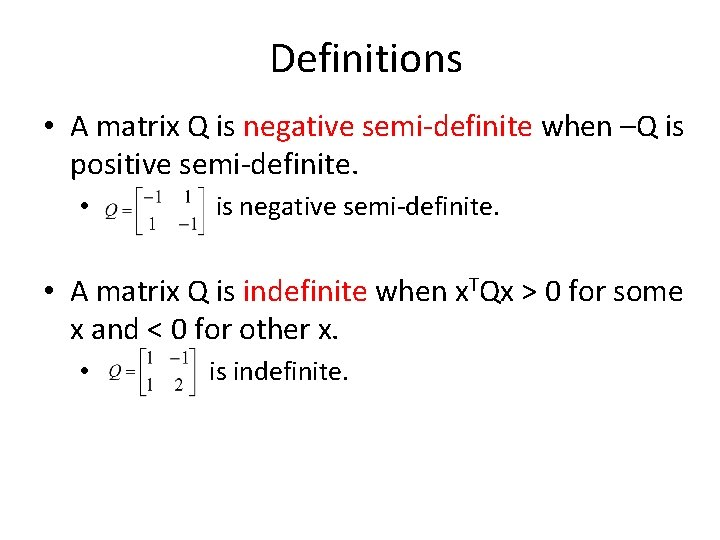Definitions • A matrix Q is negative semi-definite when –Q is positive semi-definite. • is negative semi-definite. • A matrix Q is indefinite when x. TQx > 0 for some x and < 0 for other x. • is indefinite.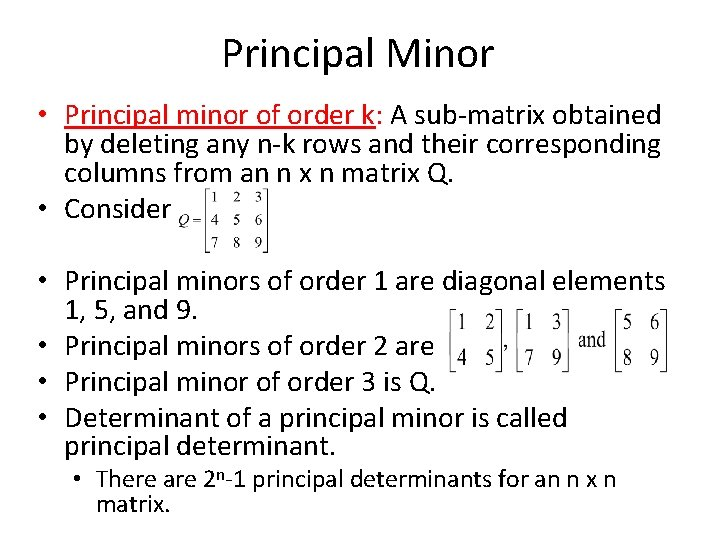Principal Minor • Principal minor of order k: A sub-matrix obtained by deleting any n-k rows and their corresponding columns from an n x n matrix Q. • Consider • Principal minors of order 1 are diagonal elements 1, 5, and 9. • Principal minors of order 2 are • Principal minor of order 3 is Q. • Determinant of a principal minor is called principal determinant. • There are 2 n-1 principal determinants for an n x n matrix.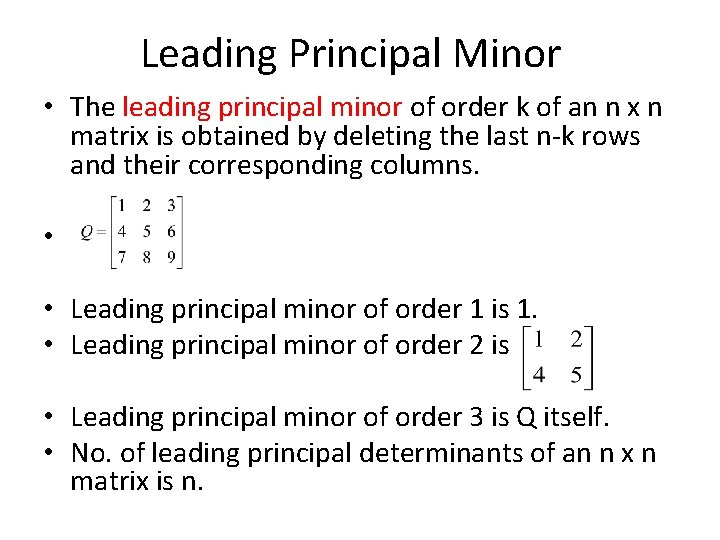Leading Principal Minor • The leading principal minor of order k of an n x n matrix is obtained by deleting the last n-k rows and their corresponding columns. • • Leading principal minor of order 1 is 1. • Leading principal minor of order 2 is • Leading principal minor of order 3 is Q itself. • No. of leading principal determinants of an n x n matrix is n.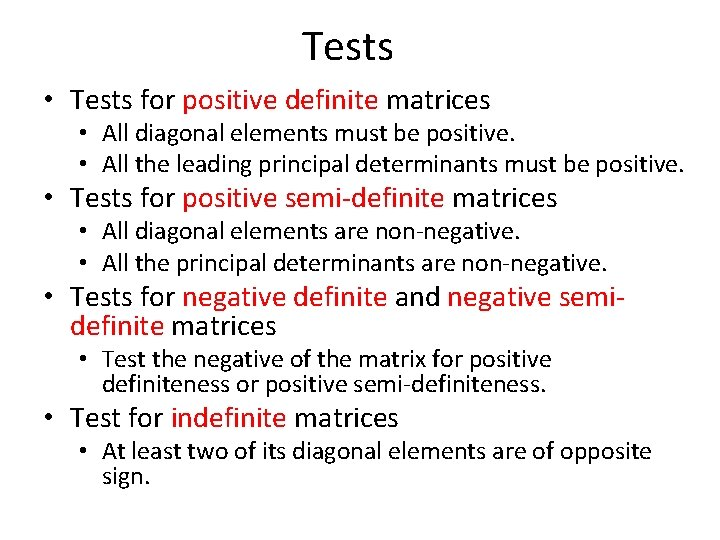Tests • Tests for positive definite matrices • All diagonal elements must be positive. • All the leading principal determinants must be positive. • Tests for positive semi-definite matrices • All diagonal elements are non-negative. • All the principal determinants are non-negative. • Tests for negative definite and negative semidefinite matrices • Test the negative of the matrix for positive definiteness or positive semi-definiteness. • Test for indefinite matrices • At least two of its diagonal elements are of opposite sign.Convex Sets • A set S is convex set if for any two points in the set the line joining those two points is also in the set. • S is a convex set if for any two vectors x(1) and x(2) in S, the vector x = λ x(1) +(1 -λ) x(2) is also in S for λ between 0 and 1.Convex Sets • The intersection of convex sets is a convex set (Figure A. 4). • The union of convex sets is not necessarily a convex set (Figure A. 4).Convex Sets • A convex combination of vectors x(1) , x(2), …. , x(n) is a vector x such that • x = λ 1 x(1)+λ 2 x(2)+…+λkx(k) • λ 1+λ 2+…+λk = 1 • λi≥ 0 for i = 1, 2, …. , k • Extreme point: A point in convex set that cannot be expressed as the midpoint of any two points in the set • Convex set S = {(x 1 , x 2)|0≤x 1≤ 2, 0≤x 2≤ 2} • This set has four extreme points (0, 0), (0, 2), (2, 0) and (2, 2) • A hyperplane is a convex set.APPENDIX B: CONVEX AND CONCAVE FUNCTIONSConvex Function • Convex function: A function is convex on set D if and only if for any two points x(1) and x(2) Є D and 0 ≤ λ ≤ 1, • f[λx(1) + (1 -λ)x(2)] ≤ λf(x(1) ) +(1 - λ) x(2)Properties of Convex FunctionsProperty 4 • The linear approximation of f(x) at any point in the interval always underestimates the true function value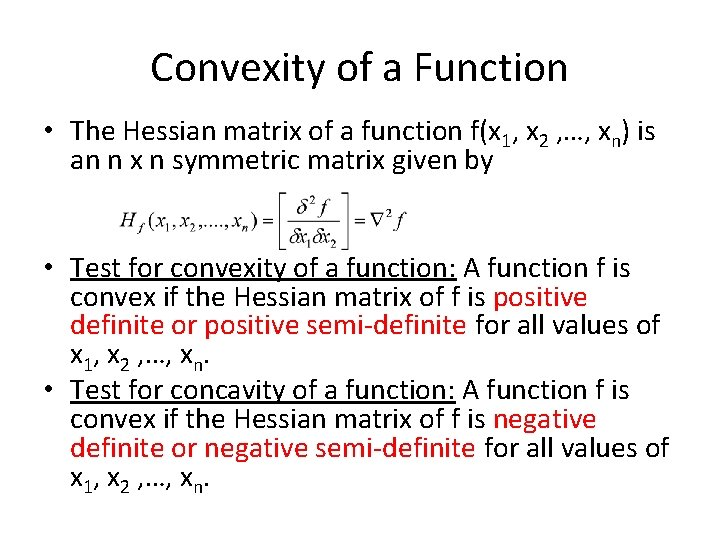Convexity of a Function • The Hessian matrix of a function f(x 1, x 2 , …, xn) is an n x n symmetric matrix given by • Test for convexity of a function: A function f is convex if the Hessian matrix of f is positive definite or positive semi-definite for all values of x 1, x 2 , …, xn. • Test for concavity of a function: A function f is convex if the Hessian matrix of f is negative definite or negative semi-definite for all values of x 1, x 2 , …, xn.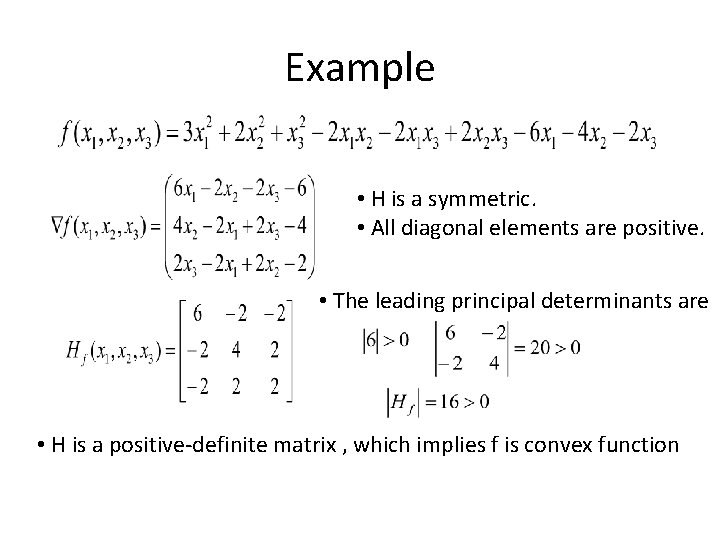Example • H is a symmetric. • All diagonal elements are positive. • The leading principal determinants are • H is a positive-definite matrix , which implies f is convex function